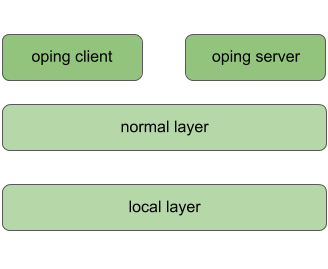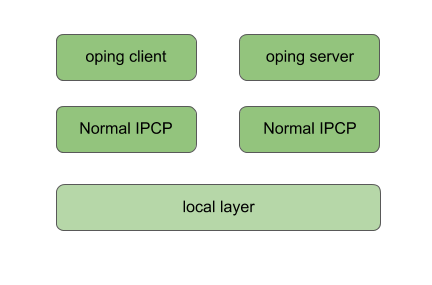# Tutorial 2: Adding a layer

In this tutorial we will add a normal layer on top of the local layer. Make sure you have a local layer running. The network will look like this:Let’s start adding the normal layer. We will first bootstrap a normal IPCP, with name “normal_1” into the “normal_layer” (using default options). In terminal 2, type:

``````\$ irm ipcp bootstrap type normal name normal_1 layer normal_layer
``````

The IRMd and IPCP will report the bootstrap:

``````==02301== irmd(II): Created IPCP 4363.
==04363== normal-ipcp(DB): IPCP got address 465922905.
==04363== directory(DB): Bootstrapping directory.
==04363== directory(II): Directory bootstrapped.
==04363== normal-ipcp(DB): Bootstrapped in layer normal_layer.
==02301== irmd(II): Bootstrapped IPCP 4363 in layer normal_layer.
==02301== irmd(DB): New instance (4363) of ipcpd-normal added.
==02301== irmd(DB): This process accepts flows for:
``````

The new IPCP has pid 4363. It also generated an address for itself, 465922905. Then it bootstrapped a directory. The directory will map registered names to an address or a set of addresses. In the normal DHT the current default (and only option) for the directory is a Distributed Hash Table (DHT) based on the Kademlia protocol, similar to the DHT used in the mainline BitTorrent as specified by the BEP5. This DHT will use the hash algorithm specified for the layer (default is 256-bit SHA3) instead of the SHA1 algorithm used by Kademlia. Just like any Ouroboros-capable process, the IRMd will notice a new instance of the normal IPCP. We will now bind this IPCP to some names and register them in the local_layer:

``````\$ irm bind ipcp normal_1 name normal_1
\$ irm bind ipcp normal_1 name normal_layer
\$ irm register name normal_1 layer local_layer
\$ irm register name normal_layer layer local_layer
``````

The “irm bind ipcp” call is a shorthand for the “irm bind proc” call that uses the ipcp name instead of the pid for convenience. Note that we have bound the same process to two different names. This is to allow enrollment using a layer name (anycast) instead of a specific ipcp_name. The IRMd and local IPCP should log the following, just as in tutorial 1:

``````==02301== irmd(II): Bound process 4363 to name normal_1.
==02301== irmd(II): Bound process 4363 to name normal_layer.
==02324== ipcpd-local(II): Registered e9504761.
==02301== irmd(II): Registered normal_1 in local_layer as e9504761.
==02324== ipcpd-local(II): Registered f40ee0f0.
==02301== irmd(II): Registered normal_layer in local_layer as
f40ee0f0.
``````

We will now create a second IPCP and enroll it in the normal_layer. Like the “irm ipcp bootstrap command”, the “irm ipcp enroll” command will create the IPCP if an IPCP with that name does not yet exist in the system. An “autobind” option is a shorthand for binding the IPCP to the IPCP name and the layer name.

``````\$ irm ipcp enroll name normal_2 layer normal_layer autobind
``````

The activity is shown by the output of the IRMd and the IPCPs. Let’s break it down. First, the new normal IPCP is created and bound to its process name:

``````==02301== irmd(II): Created IPCP 13569.
==02301== irmd(II): Bound process 13569 to name normal_2.
``````

Next, that IPCP will enroll with an existing member of the layer “normal_layer”. To do that it first allocates a flow over the local layer:

``````==02324== ipcpd-local(DB): Allocating flow to f40ee0f0 on fd 64.
==02301== irmd(DB): Flow req arrived from IPCP 2324 for f40ee0f0.
==02301== irmd(II): Flow request arrived for normal_layer.
==02324== ipcpd-local(II): Pending local allocation request on fd 64.
==02301== irmd(II): Flow on port_id 0 allocated.
==02324== ipcpd-local(II): Flow allocation completed, fds (64, 65).
==02301== irmd(II): Flow on port_id 1 allocated.
``````

Over this flow, it connects to the enrollment component of the normal_1 IPCP. It sends some information that it will speak the Ouroboros Enrollment Protocol (OEP). Then it will receive boot information from normal_1 (the configuration of the layer that was provided when we bootstrapped the normal_1 process), such as the hash it will use for the directory. It signals normal_1 that it got the information so that normal_1 knows this was successful. It will also get an address. After enrollment is complete, both normal_1 and normal_2 will be ready to accept incoming flows:

``````==13569== connection-manager(DB): Sending cacep info for protocol OEP to
fd 64.
==13569== enrollment(DB): Getting boot information.
==02301== irmd(DB): New instance (4363) of ipcpd-normal added.
==02301== irmd(DB): This process accepts flows for:
==02301== irmd(DB): normal_layer
==02301== irmd(DB): normal_1
==04363== enrollment(DB): Enrolling a new neighbor.
==04363== enrollment(DB): Sending enrollment info (49 bytes).
==13569== enrollment(DB): Received enrollment info (49 bytes).
==13569== normal-ipcp(DB): IPCP got address 416743497.
==04363== enrollment(DB): Neighbor enrollment successful.
==02301== irmd(DB): New instance (13569) of ipcpd-normal added.
==02301== irmd(DB): This process accepts flows for:
==02301== irmd(DB): normal_2
``````

Now that the member is enrolled, normal_1 and normal_2 will deallocate the flow over which it enrolled and signal the IRMd that the enrollment was successful:

``````==02301== irmd(DB): Partial deallocation of port_id 0 by process
13569.
==02301== irmd(DB): Partial deallocation of port_id 1 by process 4363.
==02301== irmd(II): Completed deallocation of port_id 0 by process
2324.
==02301== irmd(II): Completed deallocation of port_id 1 by process
2324.
==02324== ipcpd-local(II): Flow with fd 64 deallocated.
==02324== ipcpd-local(II): Flow with fd 65 deallocated.
==13569== normal-ipcp(II): Enrolled with normal_layer.
==02301== irmd(II): Enrolled IPCP 13569 in layer normal_layer.
``````

Now that normal_2 is a full member of the layer, the irm tool will complete the autobind option and bind normal_2 to the name “normal_layer” so it can also enroll new members.

``````==02301== irmd(II): Bound process 13569 to name normal_layer.
``````At this point, have two enrolled members of the normal_layer. What we need to do next is connect them. We will need a management flow, for the management network, which is used to distribute point-to-point information (such as routing information) and a data transfer flow over which the layer will forward traffic coming either from higher layers or internal components (such as the DHT and flow allocator). They can be established in any order, but it is recommended to create the management network first to achieve the minimal setup times for the network layer:

``````\$ irm ipcp connect name normal_2 dst normal_1 comp mgmt
\$ irm ipcp connect name normal_2 dst normal_1 comp dt
``````

The IPCP and IRMd log the flow and connection establishment:

``````==02301== irmd(DB): Connecting Management to normal_1.
==02324== ipcpd-local(DB): Allocating flow to e9504761 on fd 64.
==02301== irmd(DB): Flow req arrived from IPCP 2324 for e9504761.
==02301== irmd(II): Flow request arrived for normal_1.
==02324== ipcpd-local(II): Pending local allocation request on fd 64.
==02301== irmd(II): Flow on port_id 0 allocated.
==02324== ipcpd-local(II): Flow allocation completed, fds (64, 65).
==02301== irmd(II): Flow on port_id 1 allocated.
==13569== connection-manager(DB): Sending cacep info for protocol LSP to
fd 64.
==04363== link-state-routing(DB): Type mgmt neighbor 416743497 added.
==02301== irmd(DB): New instance (4363) of ipcpd-normal added.
==02301== irmd(DB): This process accepts flows for:
==02301== irmd(DB): normal_layer
==02301== irmd(DB): normal_1
==13569== link-state-routing(DB): Type mgmt neighbor 465922905 added.
==02301== irmd(II): Established Management connection between IPCP 13569
and normal_1.
``````

The IPCPs established a management flow between the link-state routing components (currently that is the only component that needs a management flow). The output is similar for the data transfer flow, however, creating a data transfer flow triggers some additional activity:

``````==02301== irmd(DB): Connecting Data Transfer to normal_1.
==02324== ipcpd-local(DB): Allocating flow to e9504761 on fd 66.
==02301== irmd(DB): Flow req arrived from IPCP 2324 for e9504761.
==02301== irmd(II): Flow request arrived for normal_1.
==02324== ipcpd-local(II): Pending local allocation request on fd 66.
==02301== irmd(II): Flow on port_id 2 allocated.
==02324== ipcpd-local(II): Flow allocation completed, fds (66, 67).
==02301== irmd(II): Flow on port_id 3 allocated.
==13569== connection-manager(DB): Sending cacep info for protocol dtp to
fd 65.
==04363== dt(DB): Added fd 65 to SDU scheduler.
==04363== link-state-routing(DB): Type dt neighbor 416743497 added.
==02301== irmd(DB): New instance (4363) of ipcpd-normal added.
==02301== irmd(DB): This process accepts flows for:
==02301== irmd(DB): normal_layer
==02301== irmd(DB): normal_1
==13569== dt(DB): Added fd 65 to SDU scheduler.
==13569== link-state-routing(DB): Type dt neighbor 465922905 added.
==13569== dt(DB): Could not get nhop for addr 465922905.
==02301== irmd(II): Established Data Transfer connection between IPCP
13569 and normal_1.
==13569== dt(DB): Could not get nhop for addr 465922905.
==13569== dht(DB): Enrollment of DHT completed.
``````

First, the data transfer flow is added to the SDU scheduler. Next, the neighbor’s address is added to the link-state database and a Link-State Update message is broadcast over the management network. Finally, if the DHT is not yet enrolled, it will try to do so when it detects a new data transfer flow. Since this is the first data transfer flow in the network, the DHT will try to enroll. It may take some time for the routing entry to get inserted to the forwarding table, so the DHT re-tries a couple of times (this is the “could not get nhop” message in the debug log).

Our oping server is not registered yet in the normal layer. Let’s register it in the normal layer as well, and connect the client:

``````\$ irm r n oping_server layer normal_layer
\$ oping -n oping_server -c 5
``````

The IRMd and IPCP will log:

``````==02301== irmd(II): Registered oping_server in normal_layer as
465bac77.
==02301== irmd(II): Registered oping_server in normal_layer as
465bac77.
==02324== ipcpd-local(DB): Allocating flow to 4721372d on fd 68.
==02301== irmd(DB): Flow req arrived from IPCP 2324 for 4721372d.
==02301== irmd(II): Flow request arrived for oping_server.
==02324== ipcpd-local(II): Pending local allocation request on fd 68.
==02301== irmd(II): Flow on port_id 4 allocated.
==02324== ipcpd-local(II): Flow allocation completed, fds (68, 69).
==02301== irmd(II): Flow on port_id 5 allocated.
==02301== irmd(DB): New instance (2337) of oping added.
==02301== irmd(DB): This process accepts flows for:
==02301== irmd(DB): oping_server
==02301== irmd(DB): Partial deallocation of port_id 4 by process 749.
==02301== irmd(II): Completed deallocation of port_id 4 by process
2324.
==02324== ipcpd-local(II): Flow with fd 68 deallocated.
==02301== irmd(DB): Dead process removed: 749.
==02301== irmd(DB): Partial deallocation of port_id 5 by process 2337.
==02301== irmd(II): Completed deallocation of port_id 5 by process
2324.
==02324== ipcpd-local(II): Flow with fd 69 deallocated.
``````

The client connected over the local layer instead of the normal layer. This is because the IRMd prefers the local layer. If we unregister the name from the local layer, the client will connect over the normal layer:

``````\$ irm unregister name oping_server layer local_layer
\$ oping -n oping_server -c 5
``````

As shown by the logs (the normal IPCP doesn’t log the flow allocation):

``````==02301== irmd(DB): Flow req arrived from IPCP 13569 for 465bac77.
==02301== irmd(II): Flow request arrived for oping_server.
==02301== irmd(II): Flow on port_id 5 allocated.
==02301== irmd(II): Flow on port_id 4 allocated.
==02301== irmd(DB): New instance (2337) of oping added.
==02301== irmd(DB): This process accepts flows for:
==02301== irmd(DB): oping_server
``````

This concludes tutorial 2. You can shut down everything or continue with tutorial 3.